# Circumference, In Terms of Pi - Set 1

### Explanation:

The circumference of a circle, "C" is the distance around a circle.  The diameter, "d" is the longest distance across a circle and passes through the center of the circle.  The radius, "r", is the distance from the circle to the center of the circle.  A diameter is made of 2 radii.  There are 2 formulas to find the circumference of a circle, Circumference = pi * diameter     and  Circumference = 2 * pi * r.  The symbol for pi is the Greek letter,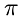, and is typically given the value of 3.14.  Leaving an answer as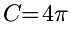is called 'leaving  the circumference in terms of pi'.  For the problems in this problem set, you will be given the radius of a circle and you are to find the circumference, leaving the answer in 'in terms of pi'.

### Example(s):

If the radius is 12: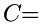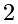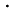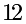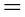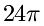### Directions:

Find the For each problem you will need to use the example above to find the circumference. Strive for accomplishing the problems with ease and then strive for speed.  Good luck and enjoy the challenge!

...